## Tuesday, January 15, 2008

### Lagrange: Lagrange's Formula

Shortly after Alexander-Theophile Vandermonde completed his paper on equations and his solution of the eleventh root of unity, Joseph-Louis Lagrange published a 200+ page work that covered much of the same material in a clearer and more authoritative manner.

Lagrange came up with the following function that returned its parameters as possible values:where

t(ω) = x1 + ωx2 + ω2x3 + ... + ωn-1xn

and

ω is an n-th root of unity

I will later use Lagrange's Formula in Gauss's general proof on cyclotomic equations.

Theorem: Lagrange's Formula

If:where

t(ω) = x1 + ωx2 + ω2x3 + ... + ωn-1xn

and

ω is an n-th root of unity

then: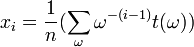Proof:

(1) From the above definition of t(ω), we have:

t(ω0) = x1 + x2 + x3 + ... + xn

t(ω1) = x1 + ωx2 + ω2x3 + ... + ωn-1xn

t(ω2) = x1 + ω2x2 + ω4x3 + ... + ω2(n-1)xn

...

t(ωn-1) = x1 + ωn-1x2 + ω2(n-1)x3 + ... + ω(n-1)2xn

(2) In general, we can see that:where i = 1 ... n

(3) Now: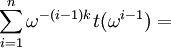(4) Now, when j ≠ k, ω(j-k) is an n-th root of unity ≠ 1 since:

(j-k)]n = ωn(j-k) = [ωn]j-k = (1)j-k = 1

(5) Since ω(j-k) is an n-th root of unity ≠ 1, and from a previous result (see Lemma 1, here), we know that:

1 + ω(j-k) + ω(j-k)2 + ... + ω(j-k)(n-1) = 0

(6) So, if j ≠ k,And, if j = k,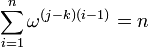(7) From step #6, it is clear that: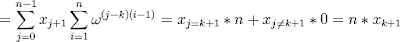(8) So, that we have: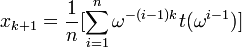(9) But ∑ (i=1,n)ω(i-1) is the same as ∑ (ω) where ω is each n-th root of unity (including 1).

(10) So that we have: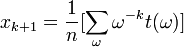(11) Adjusting for k+1 → k, gives us:QED

References